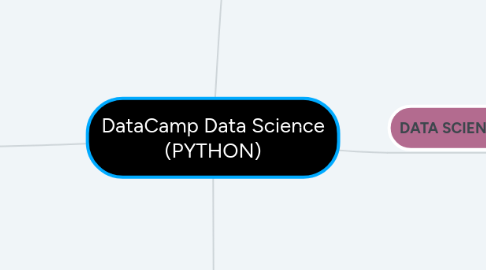# DataCamp Data Science (PYTHON)Felipe Freire

Estudo de ciência de dados com os cursos do DataCamp

Get Started. It's FreeDataCamp Data Science (PYTHON)## 1. DATA SCIENTIST

### 1.1. DATA ANALYST WITH PYTHON

1.1.1. PYTHON PROGRAMMER

1.1.1.1. PROGRAMMING

1.1.1.1.1. Introduction to Python

1.1.1.1.2. Intermediate Python for Data Science

1.1.1.1.3. Python Data Science Toolbox (Part 1)

1.1.1.1.4. Python Data Science Toolbox (Part 2)

1.1.1.2. IMPORTING & CLEANING DATA WITH PYTHON

1.1.1.2.1. Importing Data in Python (Part 1)

1.1.1.2.2. Importing Data in Python (Part 2)

1.1.1.2.3. Introduction to Databases in Python

1.1.1.2.4. Cleaning Data in Python

1.1.1.3. DATA MANIPULATION WITH PYTHON

1.1.1.3.1. Pandas Foundations

1.1.1.3.2. Manipulating DataFrames with pandas

1.1.1.3.3. Merging DataFrames with pandas

1.1.1.3.4. Analyzing Police Activity with pandas

1.1.1.4. Introduction to Relational Databases in SQL

1.1.1.4.1. DONE

1.1.1.5. Introduction to Shell for Data Science

1.1.1.5.1. DONE

1.1.1.6. Conda Essentials

1.1.1.6.1. DONE

1.1.2. Introduction to Data Science in Python

1.1.2.1. DONE

1.1.3. SQL FUNDAMENTALS

1.1.3.1. Intro to SQL for DATA SCIENCE

1.1.3.1.1. DONE

1.1.3.2. Joining Data in SQL

1.1.3.2.1. DONE

1.1.3.3. Intermediate SQL

1.1.3.4. SQL for exploratory Data Analysis

1.1.4. Introduction to Data Visualization with Python

1.1.4.1. DONE

1.1.5. Statistical Thinking in Python (Part 1)

1.1.5.1. STUDYING

1.1.6. Statistical Thinking in Python (Part 2)

### 1.3. MACHINE LEARNING

1.3.1. Supervised Learning with scikit-learn

1.3.2. Unsupervised Learning in Python

1.3.3. Linear Classifiers in Python

1.3.4. Machine Learning with Experts: School Budgets

1.3.5. Deep Learning in Python

### 2.1. PROGRAMMING

2.1.1. Python for R Users

2.1.2. Python for MATLAB Users

2.1.4. Writting Efficient Python Code

2.1.5. Object-Oriented Programming in Python

2.1.6. Data Types for Data Science

2.1.7. Working with Dates and Times in Python

2.1.8. Software Engineer for Data Scienctists in Python

### 2.2. DATA MANIPULATION

2.2.1. Manipulating Time Series Data in Python

2.2.3. Introduction to MongoDB in Python

2.2.4. Feature Engineering with PySpark

2.2.5. Biomedical Image Analysis in Python

2.2.6. Working with Geospatial Data in Python

2.2.8. Analyzing Social Media Data in Python

2.2.9. Optimizing Python Code with pandas

2.2.10. Introduction to Spark SQL with Python

### 2.3. DATA VISUALIZATION

2.3.1. Visualizing Time Series Data in Python

2.3.2. Data Visualization with Seaborn

2.3.3. Visualizing Geospatial Data in Python

2.3.4. Introduction to Matplotlib

2.3.5. Improving Your Data Visualizations in Python

### 2.4. PROBABILITY AND STATISTICS

2.4.1. Network Analysis in Python (Part 2)

2.4.2. Introduction to Time Series Analysis in Python

2.4.3. Case Studies in Statistical Thinking

2.4.4. Introduction to Linear Modeling in Python

2.4.5. Customer Analytics & A/B Testing in Python

2.4.6. Statistical Simulation in Python

### 2.5. APPLIED FINANCE

2.5.1. Importing & Managing Financial Data in Python

2.5.2. Intro to Financial Concepts using Python

2.5.3. Intro to Portfolio Risk Management in Python

2.5.4. Intro to Python for Finance

2.5.5. Financial Forecasting in Python

### 2.6. CASE STUDIES

2.6.1. Exploratory Data Analysis in Python

### 2.7. MACHINE LEARNING

2.7.1. Natural Language Processing Fundamentals in Python

2.7.2. Building Chatbots in Python

2.7.3. Extreme Gradient Boosting with XGBoost

2.7.4. Machine Learning for Time Series Data in Python

2.7.5. HR Analytics in Python: Predicting Employee Churn

2.7.6. Machine Learning with Tree-Based Models in Python

2.7.7. Foundations of Predictive Analytics in Python (Part 1)

2.7.8. Foundations of Predictive Analytics in Python (Part 2)

2.7.9. Advanced Deep Learning with Keras in Python

2.7.10. Preprocessing for Machine Learning in Python

2.7.11. Machine Learning for Finance in Python

2.7.12. Building Recommendation Egines with PySpark

2.7.13. Convolutional Neural Networks for Image Processing

2.7.14. Supply Chain Analytics in Python

2.7.15. Fraud Detection in Python

2.7.16. Customer Segmentation in Python

2.7.17. Bid Data Fundamentals via PySpark

2.7.18. Dimensionality Reduction in Python

2.7.19. Designing Machine Learning Workflows in Python

2.7.20. Cluestering Methods with SciPy

2.7.21. Model Validation in Python

### 2.8. OTHER

2.8.1. Introduction to PySpark

2.8.2. Web Scraping in Python